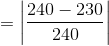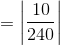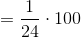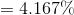# College Chemistry : Yield and Error

## Example Questions

### Example Question #1 : Yield And Error

The literature value for the melting point of a given compound is 240 degrees Celsius. A scientist uses the melting point apparatus to experimentally measure the compound's melting point at 230 degrees Celsius. What is the scientist's percent error?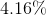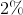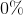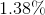Explanation:

The percent error is given by the following formula: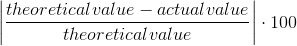In this case, the theoretical value is 240 degrees Celsius, and the actual value is 230 degrees Celsius. The percent error is thus calculated to be 4.16 %. The calculation is as follows: